Trigonometric Equations

Ex 1 Solve the equationfor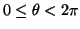.

Sol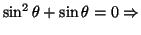a)or b), so a)oror b)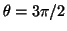.

Ex 2 Find all solutions of the equation.

Solgives; andfor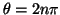(where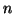is any integer) and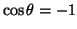for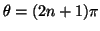(whereis any integer).

Combining these solutions gives that, where k is any integer.

Pr A Find all solutions of the equation.

Pr B Solve the equationfor.

Pr C Solve the equation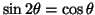for.

Pr 1 Solve the equationfor.

Pr 2 Solve the equationfor.

Pr 3 Solve the equationfor.

Pr 4 Find all solutions of the equation.

Pr 5 Solve the equationfor.

Pr 6 Solve the equation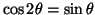for.

Pr 7 Solve the equation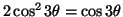for.

Pr 8 Solve the equationfor.

Lawrence Marx 2004-04-09